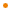# JavaFX: Bringing Rich Experiences To All the Screens Of Your Life

### Overview

A 3D geometric point that represents the x, y, z coordinates.

Profile: common conditional scene3d

### Variable Summary

accessnametypedescription
public-initxNumber0.0

The x coordinate.

public-inityNumber0.0

The y coordinate.

public-initzNumber0.0

The z coordinate.

### Function Summary

public distance(x1: Number, y1: Number, z1: Number) : Number

Computes the distance between this point and point `(x1, y1, z1)`.Computes the distance between this point and point `(x1, y1, z1)`.

Parameters
x1
the x coordinate of other point
y1
the y coordinate of other point
z1
the z coordinate of other point
Returns
Number
the distance between this point and point `(x1, y1, z1)` .

Profile: common conditional scene3d

public distance(p: Point3D) : Number

Computes the distance between this point and point `p`.Computes the distance between this point and point `p`.

Parameters
p
the other point
Returns
Number
the distance between this point and point `p` .

Profile: common conditional scene3d

public equals(obj: java.lang.Object) : BooleanParameters
obj
Returns
Boolean

public hashCode() : IntegerReturns
Integer

public toString() : java.lang.String

Returns a string representation of this `Point3D`.Returns a string representation of this `Point3D`. This method is intended to be used only for informational purposes. The content and format of the returned string might vary between implementations. The returned string might be empty but cannot be `null`.

Returns
String

Profile: common conditional scene3d